# six-fold symmetry with hexagons

The remaining patterns are numbered 187, which implies that one more further question needs to the answered.

The first group are examples of patterns with equilateral triangles. An example of two of these are: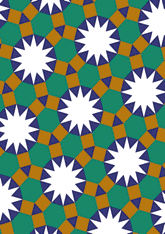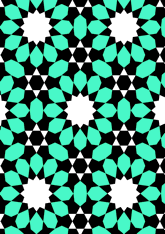The number in this group is 46, which is 24% of the total. Another question is needed - for this see page.
The second group are examples of patterns without equilateral triangles. An example of two of these are: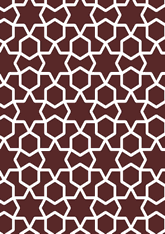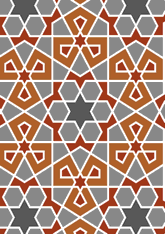The number in this group is 68, which is 36% of the total. Another question is needed - for this see page.
The third group are examples of patterns which have both of the two types of stars. An example of two of these are: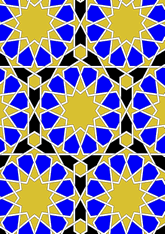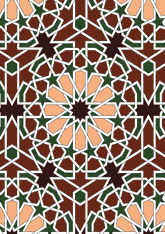The number in this group is 32, which is 17% of the total. Another question is needed - for this see page.
The last group are examples of patterns with none of the above. An example of two of these are: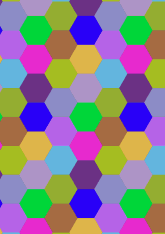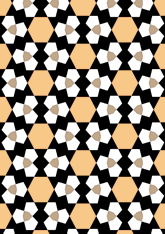The number in this group is 41, which is 21% of the total. Another question is needed - for this see page.

v53

Tiling Search was created by Brian Wichmann, and is maintained by MIT Libraries, through support from the Aga Khan Documentation Center.

Also supported by Performant Software Solutions LLC.